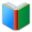# List 1 for 8th Grade MATH

Middle School Words: List 1 for 8th Grade MATH, all words and explanations of the list are in one page. You can download and print the worksheet from your browser directly.
 Free Online Vocabulary TestK12, SAT, GRE, IELTS, TOEFL
 Actions upon current list

 All lists of current grdae
Spelling Words:

Arts Words:

Social Words:

Math Words:

Science Words:

Literature Words:Animal FarmThe Hound of the Baskervilles
 Worksheet - Middle School Words: List 1 for 8th Grade MATH

 Print: List 1 for 8th Grade MATH

Exam Word - https://www.examword.com/

Middle School Words: List 1 for 8th Grade MATH

 adjacent anglesare angles that have a common ray coming out of the vertex going between two other rays, with no overlap of the regions coefficienta constant number that serves as a measure of some property or characteristic constantinvariable; repeating; continually occurring; persistent distance formulaalgebraic expression that gives the distance between two points in a particular coordinate system in a particular number of dimensions domainfield; territory over which rule or control is exercised; networked computers that share a common address formulaplan; directions for making something; a group of symbols that make a mathematical statement hypotenusethe side of a right triangle opposite the right angle lateral areathe sum of the areas of the lateral (vertical) faces of a cylinder, cone, frustum, or the like linear equationa polynomial equation of the first degree linear inequalityan inequality which involves a linear function. Pythagorean theorema relation in Euclidean geometry among the three sides of a right triangle, it states that the square of the hypotenuse is equal to the sum of the squares of the other two sides range of a functionthe set of values of the dependent variable for which a function is defined scatter plota type of display using Cartesian coordinates to display values for two variables for a set of data scientific notationa method of writing or displaying numbers in terms of a decimal number between 1 and 10 multiplied by a power of 10 slopebe at an angle; incline; gradient slope-intercept formthe equation of a straight line in the form y = mx b where m is the slope of the line and b is its y-intercept solidsgeometric figure having three dimensions surface areathe extent of a 2-dimensional surface enclosed within a boundary termlimited period of time; point in time at which something ends; termination; deadline